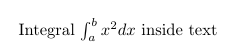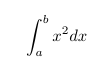## Integrals

Integral expression can be added using the

\int_{lower}^{upper}


command.

Note, that integral expression may seems a little different in inline and display math mode - in inline mode the integral symbol and the limits are compressed.

LaTeX code Output
Integral $\int_{a}^{b} x^2 dx$ inside text$$\int_{a}^{b} x^2 dx$$## Multiple integrals

To obtain double/triple/multiple integrals and cyclic integrals you must use amsmath and esint (for cyclic integrals) packages.

LaTeX code Output